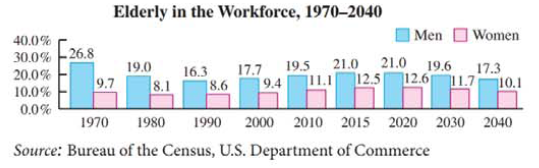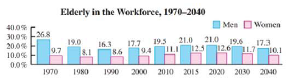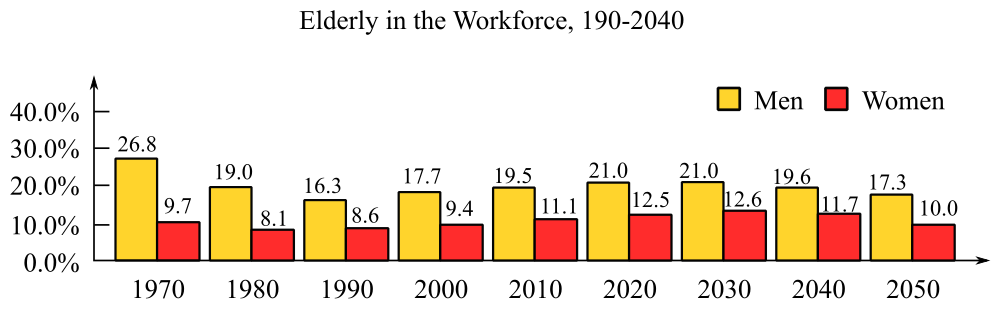Chapter 9, Problem 89RE### Mathematical Applications for the ...

11th Edition
Ronald J. Harshbarger + 1 other
ISBN: 9781305108042

#### Solutions

Chapter
Section### Mathematical Applications for the ...

11th Edition
Ronald J. Harshbarger + 1 other
ISBN: 9781305108042
Textbook Problem

# Elderly in the workforce The graph shows the percent of elderly men and women in the workforce for selected years from 1970 and projected to 2040. Use this graph in Problems 89 and 90.For the period from 1990 to 2030, find and interpret the annual average rate of change of(a) elderly men in the workforce.(b) elderly women in the workforce.(a)

To determine

To calculate: The average rate of change of elderly men, over the period 1990 to 2030 and what it means, given by the graph which represents the percent of elderly men and women in the workforce,Explanation

Given Information:

The provided graph represents the percent of elderly men and women in the workforce from the year 1970 to 2040,

Formula used:

The average rate of change of a function y=f(x) from x=a to x=b is defined by

Average rate of change=f(b)f(a)ba

Calculation:

Consider the provided graph,

For the period 1990 to 2030, the values of the function at x=1990 and x=2030 are found from the table.

f(1990)=16.3f(2030)=21

(b)

To determine

To calculate: The average rate of change of elderly women, over the period 1990 to 2030 and what is means, given by the graph which represents the percent of elderly men and women in the workforce,### Still sussing out bartleby?

Check out a sample textbook solution.

See a sample solution

#### The Solution to Your Study Problems

Bartleby provides explanations to thousands of textbook problems written by our experts, many with advanced degrees!

Get Started

#### Sketch the graphs of the equations in Exercises 512. 2y+x=1

Finite Mathematics and Applied Calculus (MindTap Course List)

#### In Exercises 2528. solve the equation by factoring. 28. 2x4 + x2 = 1

Applied Calculus for the Managerial, Life, and Social Sciences: A Brief Approach

#### Evaluate the indefinite integral. ex1+exdx

Single Variable Calculus: Early Transcendentals

#### limx0cscx= a) 0 b) c) d) does not exist

Study Guide for Stewart's Single Variable Calculus: Early Transcendentals, 8th